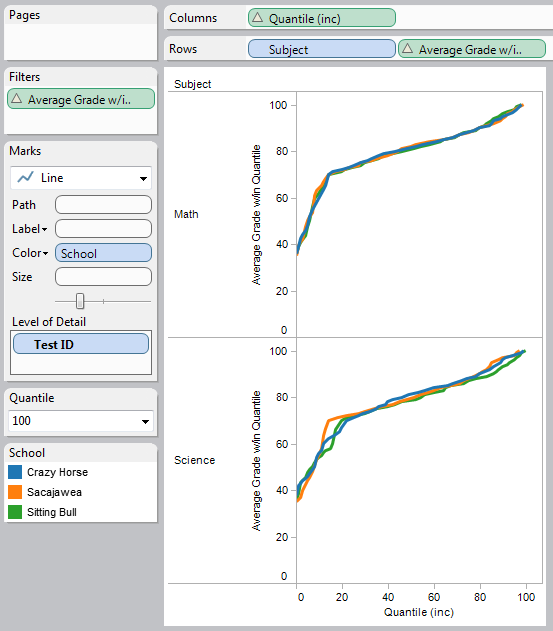# Partitioning by Table Calculations

Here’s a issue on the Tableau forums that shows up at least once per month or so: someone has a data set that they want to compute a measure over, and the measure requires table calculations. Then they want to use the results of those calculations as a discrete value for partitioning other calculations. For example, given a set of student grades, compute each student’s percentile, then show the average of each percentile in the same view. Or a similar idea, only the percentile is based on sum of sales, and you want to show the top 10% and bottom 10% of sales.

Based on the feedback I’ve gotten, it’s clear that I skipped over some steps and concepts in this tutorial. Continue on at your own risk until I get an update…

The problem here is that Tableau does not support partitioning table calculations by the results of other table calculations. You can partition table calculations by dimensions, and even by discrete aggregates if you turn off Ignore Table Calculations using the pill’s menus, but not by table calculations. An alternative workaround is to do the percentile calc in one worksheet, export the data, then use that as a datasource for an additional worksheet, but that has the limitations of a) not being dynamic and b) prone to failure for datasets in the millions of rows.

Vote for the Partition Table Calculations by Other Table Calculations feature request if you want to be able to skip this tutorial in the future. Also, the particular example I’m using with percentiles probably wouldn’t be necessary if there was a native PERCENTILE function in Tableau, since we could likely then use those results for partitioning additional calcs. And there’s even an Idea to automate the workaround of using a table worksheet as a datasource. Finally, if you’re fuzzy on the concept of partitions, here’s a bunch of links on learning table calculations.

However, table calculations are magical things that can slice, dice, rearrange, re-aggregate, and yes, Martha, even partition by the results of other table calculations, and you’re about to learn how. Thanks to Joe Mako for his pioneering work, it led me to a Tableau epiphany that I’ll be sharing in a future post.

## The Method

Here’s the basic process for partitioning the results of a table calculation by other table calculations:

1. Start with a text table. Put all the dimensions and discrete aggregates you’ll need for the final view on the Rows Shelf, with the addressing pill(s) on the right, partitioning pills on the left.
2. Build the initial table calc and verify that it’s working properly.
3. Build the partitioning calc(s), add those and verify them.
4. Do the work to reduce overlapping text/additional marks.
5. Duplicate the worksheet and rearrange pills to get to the final view.

The tricky bit is step 3, since every calc that we want to use in the final result has to appropriately reference the calc we are partitioning over. For example, for doing an average of test scores within a percentile, we need to compute the total of the scores within each percentile for the numerator, and the total number of tests within each percentile for the denominator, and  then in a third calc generate the average. If we want the average of scores within a range of percentiles, then we’ll need to return the scores for the range and compute the average over that.

## Sample Data

The data set that I’m using is a bunch of test scores that I mocked up, for three schools with two subjects, here’s a sample of the data and the school data.xlsx spreadsheet: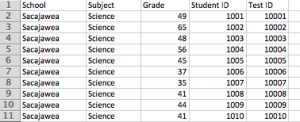For the percentile calc, I’m using Richard Leeke’s implementation of a percentile including ties from this post from the massive Table Calculation: Quantile (version 2) thread. The formula is:

```// The quantile rank gives the proportion of values the same as or less than the value in question
// Note that the term subtracting half the number of records for the current value is effectively
// returning the average rank in the case of multiple occurences of the same value.
// See http://http://en.wikipedia.org/wiki/Percentile_rank.
// Calc from http://community.tableausoftware.com/message/189088#189088
PREVIOUS_VALUE(0)
ELSE
INT([Quantile] * (RUNNING_SUM(SUM([Number of Records])) - ((SUM([Number of Records]) - 1)/ 2)) / TOTAL(SUM([Number of Records])))
END```

[Grade] is the student’s particular grade, [Quantile] is a parameter, set to 100 in this case. In order to accurately compute the percentiles, we need all the grades i.e. every row, so [Test ID] needs to be in the view, sorted by Sum of Grade/Ascending, and the Compute Using for this calc is set to Test ID.

If your data doesn’t have a dimension that uniquely identifies each row, then hopefully you can create one by concatenating several strings together. You could use a Set for the same purpose, however Sets start getting slow when they have a lot of members, and conversely Tableau can materialize the results of the concatenated string in the data source and be fast.

Here’s a starter view: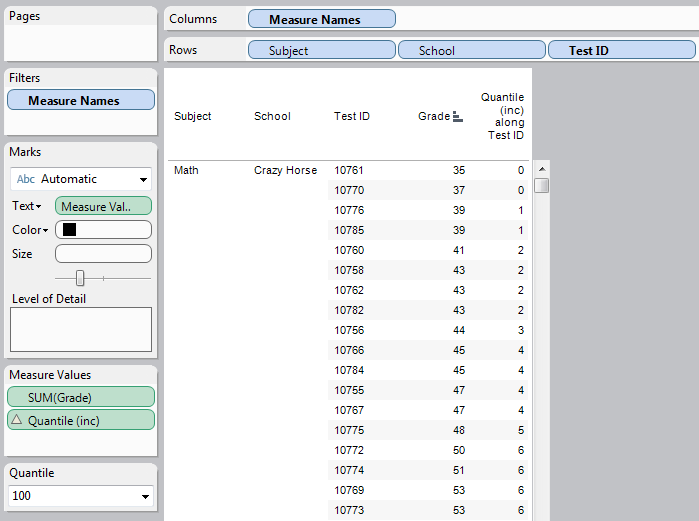## Making Friends with LOOKUP() and PREVIOUS_VALUE()

Now we can move on to the partitioning calcs. These calculations make use of two very powerful table calculation functions:

• LOOKUP([field],[offset]) gets the value of [field] from another row in the partition, based on the offset.
• PREVIOUS_VALUE([field]) is a self-referencing function, where it returns the value of the calculation from the prior row in the partition, and returns the given [field] for the first row in the partition.

Here’s the Total of Grades w/in Quantile formula, which has a Compute Using of Test ID:

```IF LOOKUP([Quantile (inc)],-1) == [Quantile (inc)] THEN
ELSE
END```

The IF statement is comparing the current row’s Quantile to the previous Quantile. If the two values are the same, then it adds the current row’s SUM([Grade]) the prior value of the Total of Grades w/in Quantile; this effectively is a running sum. Where the partitioning comes in is that if the current row’s Quantile is different, then the calculation “restarts” and returns the SUM([Grade]).

The Total Records w/in Quantile formula is even simpler, all it does is count from 1 up for each Quantile and still uses the Compute Using of Test ID:

```IF LOOKUP([Quantile (inc)],-1) == [Quantile (inc)] THEN
PREVIOUS_VALUE(0)+1
ELSE
1
END```

The Average Grade w/in Quantile uses this formula, again with a Compute Using of Test ID:

```IF LOOKUP([Quantile (inc)],1) != [Quantile (inc)]
OR LAST()==0 THEN
[Total of Grades w/in Quantile]/[Total Records w/in Quantile]
END```

The IF statement is used to identify the last row within each Quantile “partition”, and the very last row, and then return the average for that row and Null for everything else. The reason why we need the IF statement is two-fold: One is that every row has a value for the Total Grades and Total Records, but the only accurate division result is the one based on the last row within each Quantile. The second reason is that we really do only want to return one value for the average within each Quantile in order to prevent overlapping text and improve performance by drawing fewer marks.

Here’s the the basic workout view created by these calcs: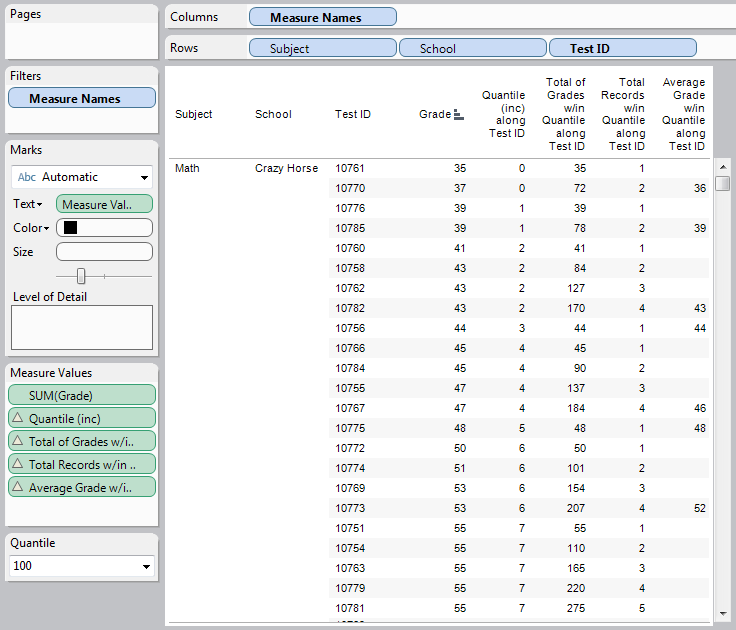Once you have that, then you can duplicate the worksheet and start rearranging pills, to create something like another text table. Note that the table has blank spaces because there aren’t test scores in the data to cover every possible quantile, and the quantile calc is not attempting to interpolate for the blanks: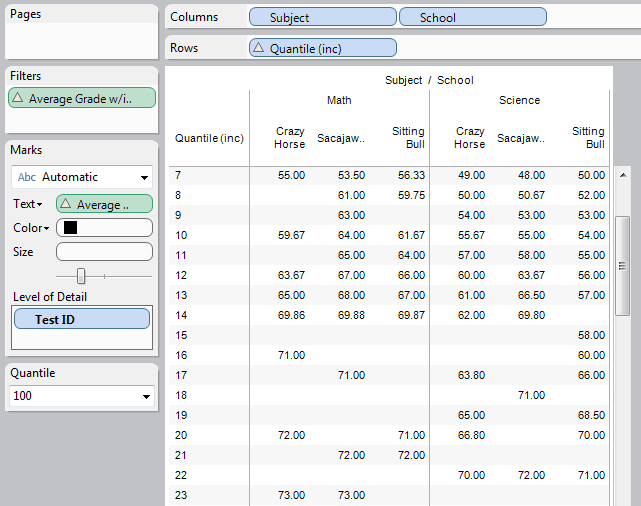Or a line chart:

In both of these views, there’s a filter on Average Grade w/in Quantile that is set to only include non-Null values. This reduces the number of marks Tableau will display and significantly improves performance when you are displaying more than a few hundred marks.

## Averaging Top 10th and Bottom 10th Percentiles

For these calculations, we again use the Quantile results as the basis for another calculation to create new measures:

```IF FIRST()==0 THEN
WINDOW_AVG(IF [Quantile (inc)] >= 91 THEN ATTR([Grade]) END,0,IIF(FIRST()==0,LAST(),0))
END```

In this case, the calc only return the Grade if the Quantile is in the top 10th percentile, then do a WINDOW_AVG of those results. A similar calc looks at the bottom 10th percentile. With those measures, we can combine them into a plot of the means and the median: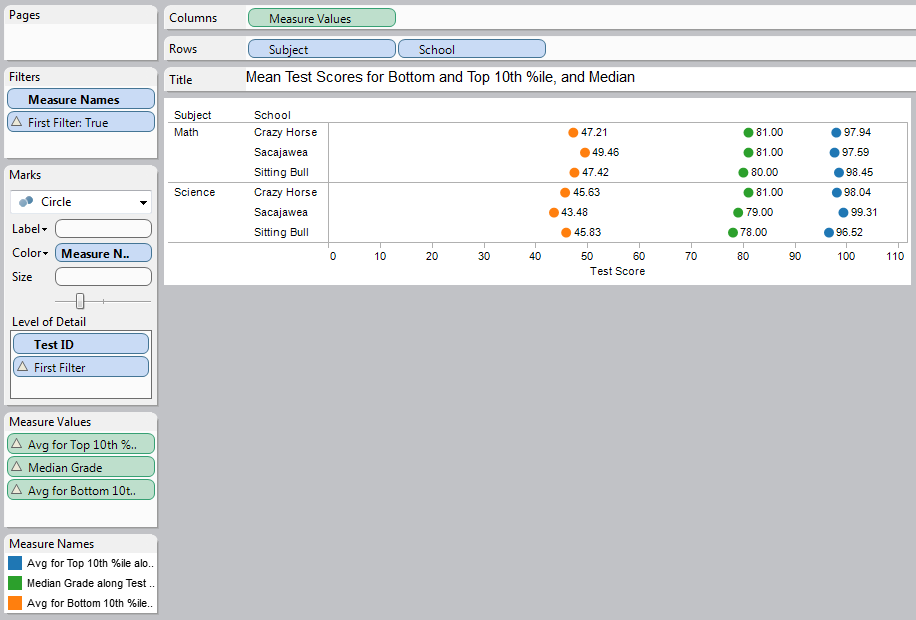There’s a basic example of how to partition table calculations by the result of other table calculations, and the partitioning by table calculations workbook on Tableau Public.

## 9 thoughts on “Partitioning by Table Calculations”

1.Jay

This is a very good walkthrough of how to do do table calcs. Sadly, I still can’t follow it completely because I can’t abstract this from the sample file to my actual situation. To your point, it seems rather annoying that I have to create basically four fields just so I can get to an aggregate value.

When I follow this technique, I can get to the final stage where I’ve got a text table with the quartile, the two interim total calculations, and the overall average for each quartile. However, I cannot then take this table and turn it into a chart. Heck, I can’t even get to the point where I have a table view that shows the average achievement per quartile. Can’t figure out what I’m doing wrong.

1.Jonathan Drummey Post author

Hi Jay

Yes, this is a hack. 🙂

Here’s one thought about why your views might not work: Have you explicitly set the Compute Using for each of the calcs and their nested child calcs? If you haven’t, then when you duplicate the working cross tab and start moving pills around the calcs will stop providing the desired results.

Shoot me an email if you’d like to get some more help on this.

Jonathan

1.Jay

One thing that was not completely clear to me in the Compute Using steps was how to set up the Compute Using of the totals fields. In my Quintile function, I am using two fields rather than your one, and I am still using the desired field as my Order Along variable. But in the two Total fields you outlined, the instructions say to use the TestID. I’m using my equivalent, but I’m wondering if I should have it match the same compute using setup as in the Quintiling function?

2.Jay

Oh, one other question I had that was not clear in your explanation – how does this technique respond to dynamic filtering within Tableau? So, for example, if the data were expanded to include a Semester and modified the quantiling to account for Semester as well, would I be able to filter for a specific Semester and have the calculations carry through correctly, or would that not work?

1.Jonathan Drummey Post author

Hi Jay,

As I’ve read through your questions, read through my post again and gotten some other feedback, it’s clear that I jumped over a number of steps along the way and need to do a revision of this post and fill in the gaps, identify what’s important to pay attention to, and where things can go off the rails.

With regards to your question about Compute Using, assuming you are trying to duplicate what I’d posted, using two dimensions for addressing shouldn’t cause any problems, so long as the Compute Using settings of the quantile calc and the calcs built on it are all the same, including both addressing and the sort. One thing to be careful of here is making sure that the nested table calcs all have the desired Compute Using settings – when a table calculation uses another table calculation, you can set the Compute Using for each in the Edit Table Calculation dialog.

Now onto the second question: Once you’ve made the Compute using for a calculation explicit, then any dimensions added to the view are automatically partitioning. So you could add Semester to the view and then the calcs would automatically return sets of quantiles, etc. within the different values of Semester. You could filter on Semesters and that wouldn’t change the results for the other Semesters. Now, if you want the table calculations to calculate across different Semesters, then Semester needs to be part of the addressing, and depending on what you want there are different options for At the Level and Restarting Every. Filtering Semesters at that point also requires a decision as to whether you want to completely filter out certain Semesters (using a regular filter), or retain the Semester’s data in the computation but “hide” the results (using a filter based on a table calculation).

I plan to do an update of this post, and I’m in the middle of getting ready for the Tableau Customer Conference next week so I probably won’t get a chance to update this for a couple of weeks. If you have any questions in the meantime, I’ll be happy to answer them as best I can and they’ll help me figure out what I need to clarify.

Jonathan

1.Jay

Jonathan – thanks for that. I appreciate it. If it makes you feel any better, your post was the first one I’ve been able to actually follow to the point where I could recreate the maths. 🙂

I will also be at the Tableau conference – perhaps I will run into you there! So many sessions to attend. I need to clone myself!

3.Sam

Can you explain the “0” Quantile?

1.Jonathan Drummey Post author

Hi Sam,

This view was set up with percentiles from 0-100, so the 0 Quantile result is the 0th percentile.

Note that the Quantile (inc) formula can be replaced wit RANK_PERCENTILE(SUM[Grade])), Tableau’s Rank functions were introduced after this post came out (though RANK_PERCENTILE() uses a slightly different algorithm).

Jonathan

Jonathan